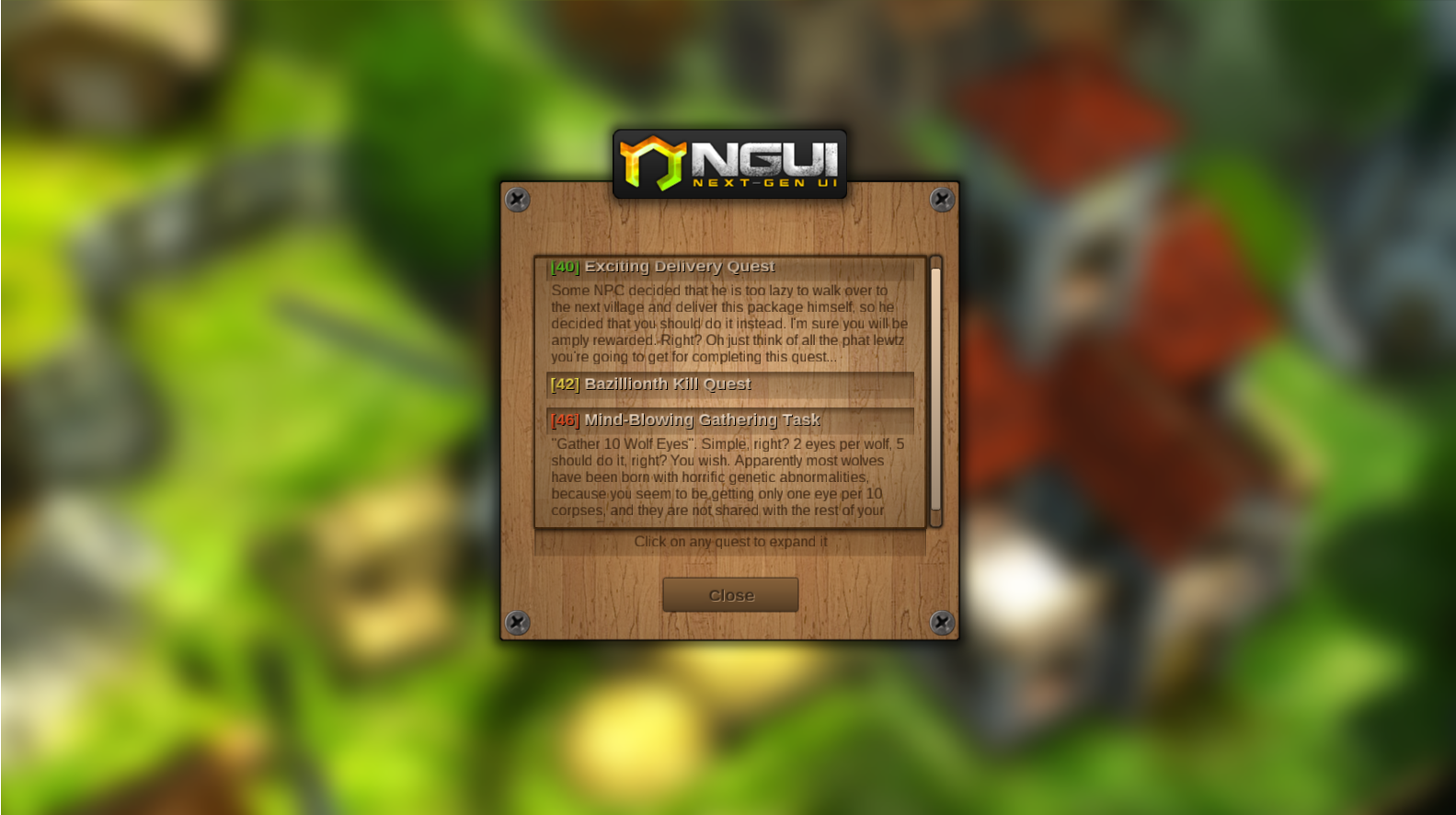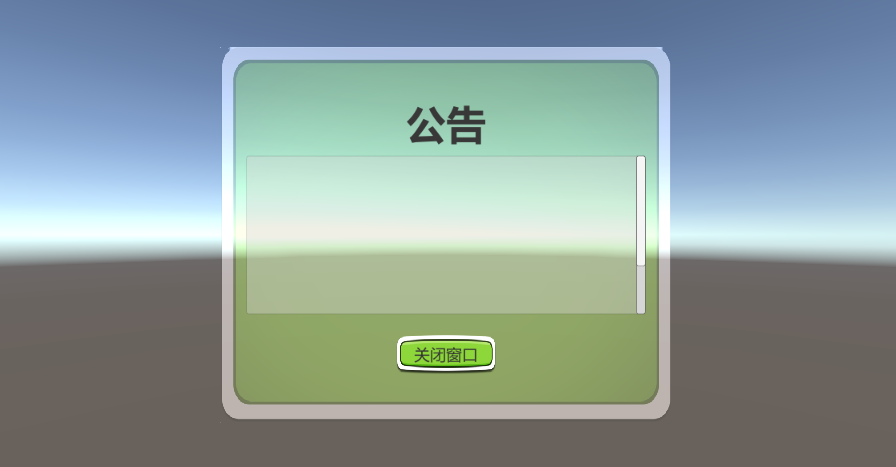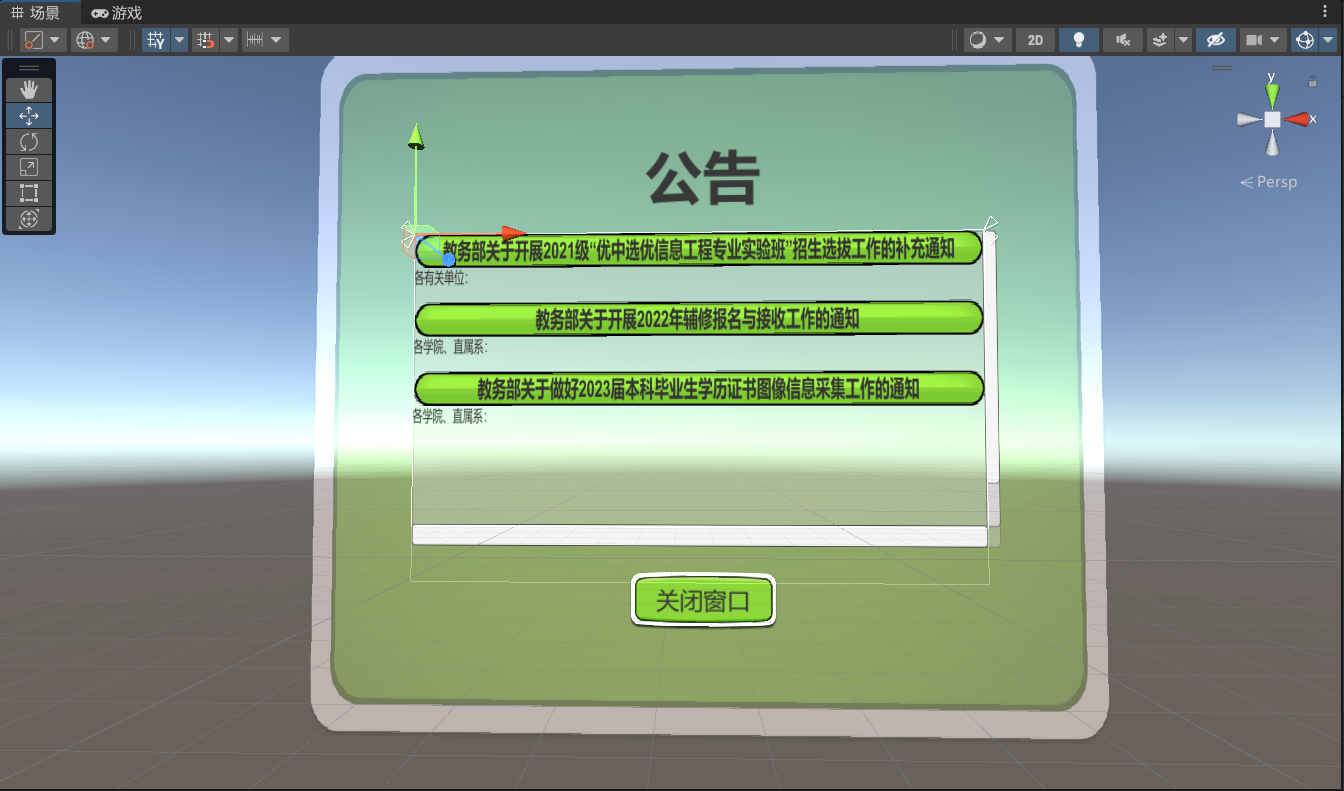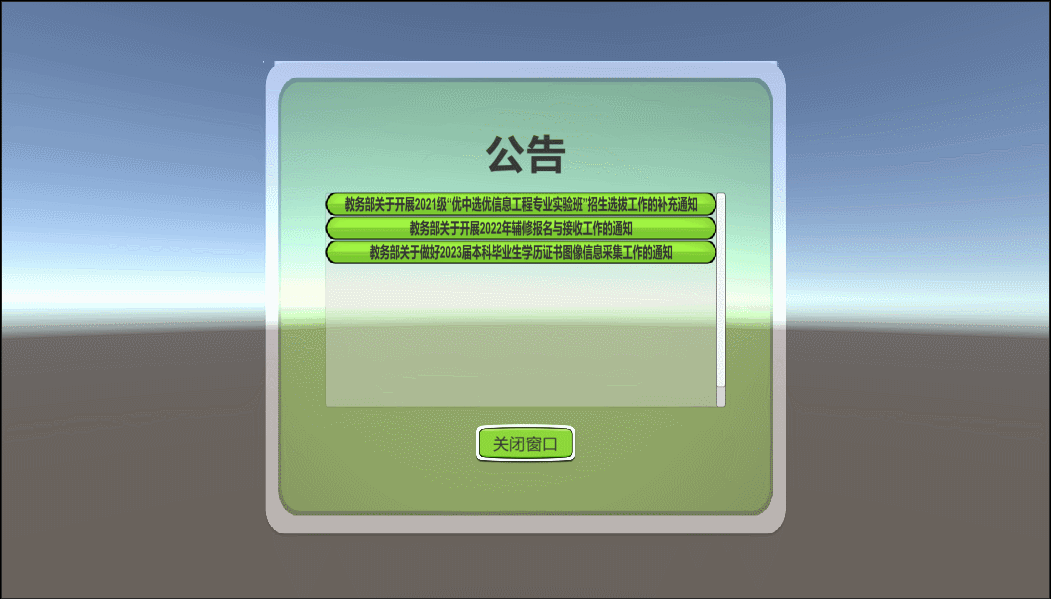# Unity3dUI系统 UI 效果制作

Inventory 背包系统
Quest Log 公告牌
Scroll View 选择板

• Windows 版 IE11
• Mac OS X 10.7 Safari

NGUI提供的示例如下图：## 场景布置## 滚动视图的完善``````using UnityEngine.UI;

public class extend : MonoBehaviour
{
public Text text;  // 控制的Text对象
private int frame = 20;  // 动画帧数

void Start()
{
Button btn = this.gameObject.GetComponent<Button>();  // 获取Button对象
text.gameObject.SetActive(false);  // 初始化时隐藏Text
}

IEnumerator FoldAndUnfold(bool isFold)
{
Vector2 fold = new Vector2(0, 120);  // 折叠状态
Vector2 unfold = new Vector2(-90, 0);  // 展开状态
Vector2 target = isFold ? fold : unfold;
for (int i = 0; i < frame; i++)  // 逐帧执行动画
{
target.x += (isFold ? -90f : 90f) / frame;  // 旋转角度逐帧增加或减少
target.y += (isFold ? -120f : 120f) / frame;  // Text高度逐帧增加或减少
text.transform.rotation = Quaternion.Euler(target.x, 0, 0);  // 设置旋转角度
text.rectTransform.sizeDelta = new Vector2(text.rectTransform.sizeDelta.x, target.y);  // 设置Text高度
if (i == 0 || i == frame - 1)  // 第一帧和最后一帧控制Text的显示状态
{
if(isFold){
text.gameObject.SetActive(false);
}
else{
text.gameObject.SetActive(true);
}
}
yield return null;
}
}

{
if (text.gameObject.activeSelf)  // 判断Text是否显示
{
StartCoroutine(FoldAndUnfold(true));  // 执行折叠动画
}
else
{
StartCoroutine(FoldAndUnfold(false));  // 执行展开动画
}
}
}``````

## 效果展示https://www.yizuodi.cn/category/3DGame/